Chapter 1 Class 9 Number Systems

Class 9
Important Questions for Exam - Class 9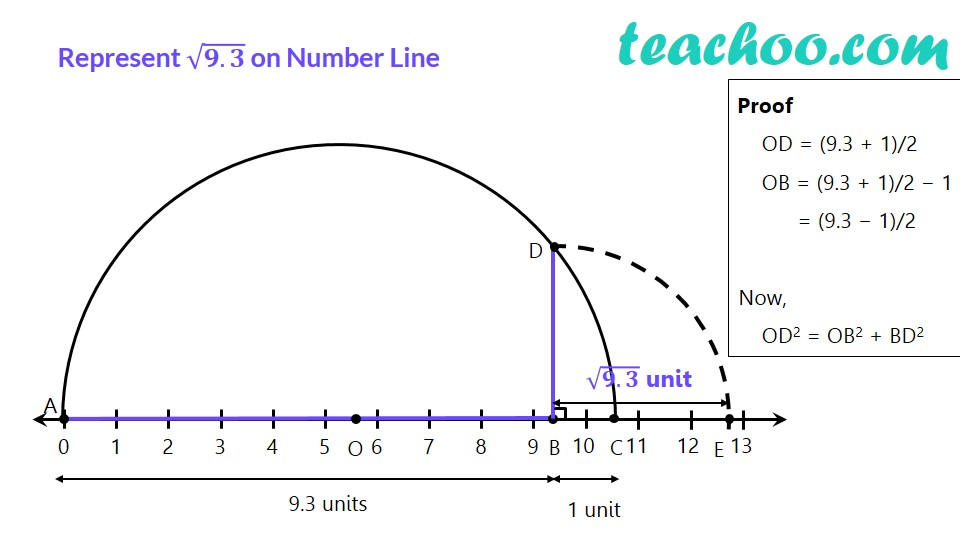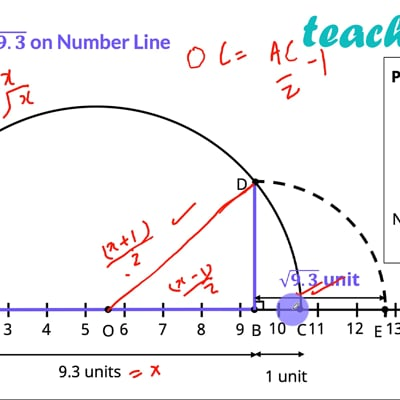This video is only available for Teachoo black users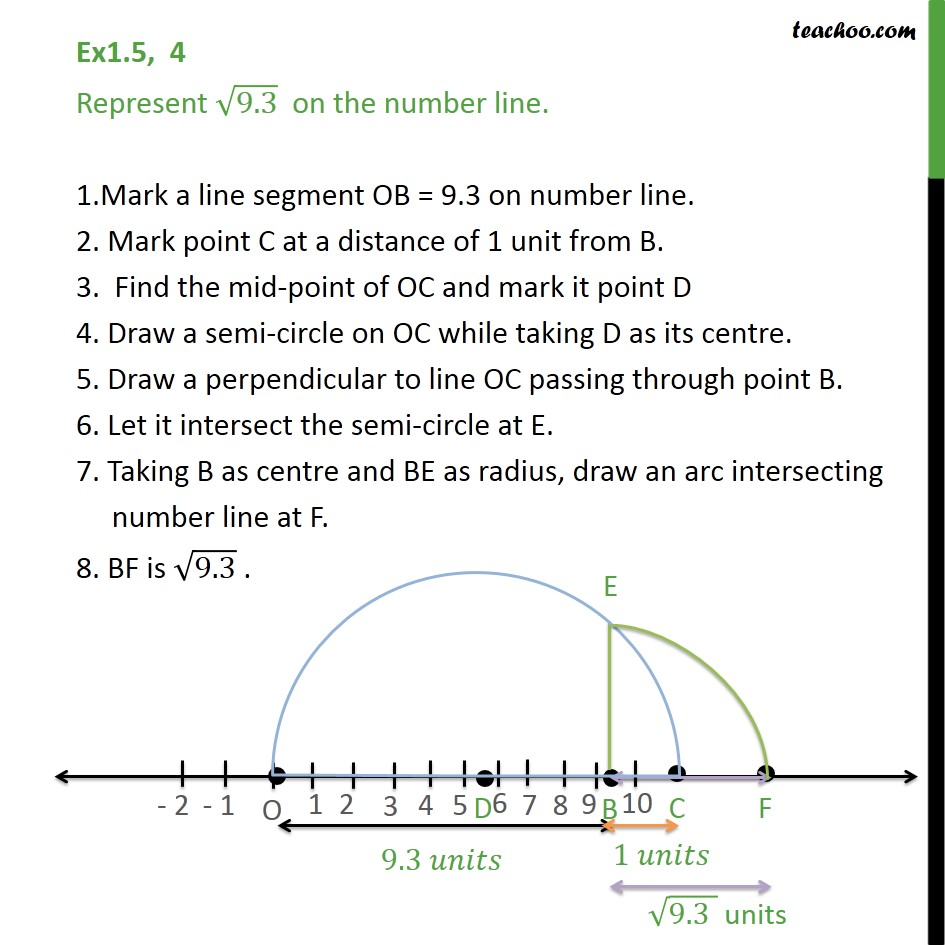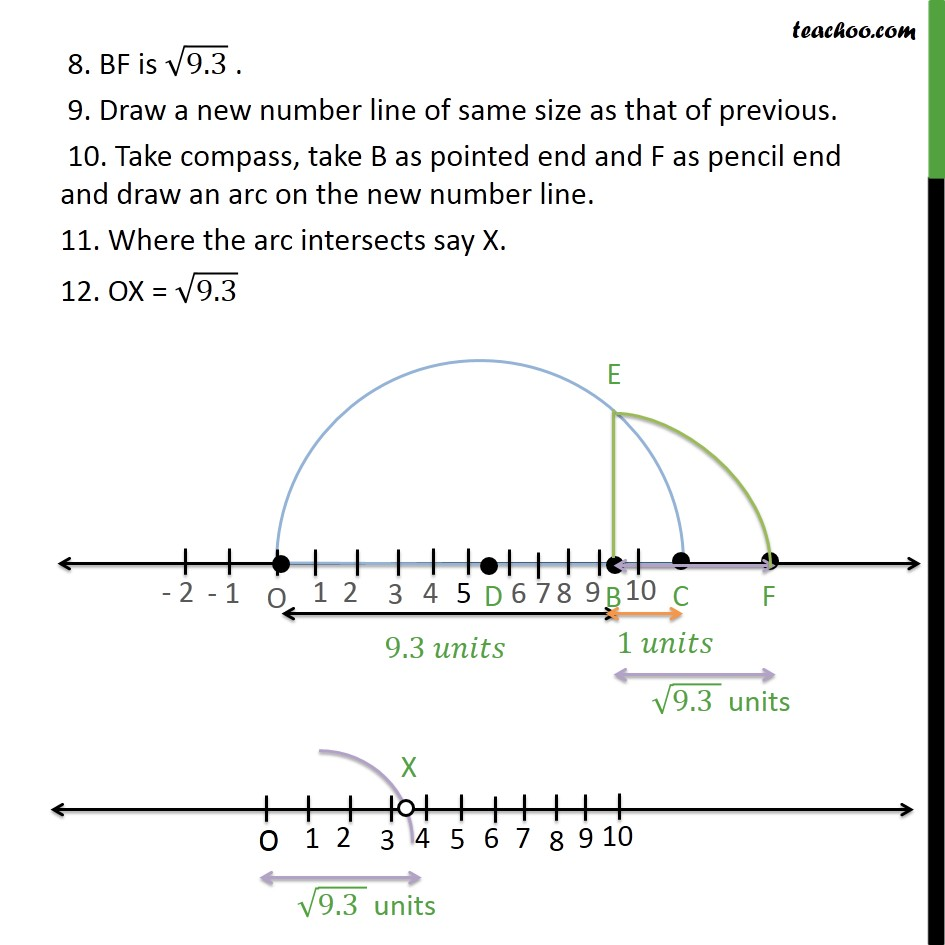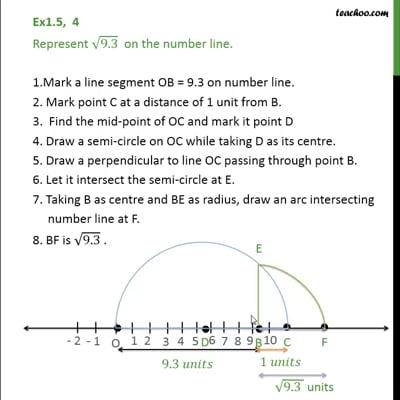This video is only available for Teachoo black users

### Transcript

√(𝟗.𝟑) & √(𝟑.𝟓) on the Number line Represent √(𝟗.𝟑) on Number Line Proof OD = (9.3 + 1)/2 OB = (9.3 + 1)/2 − 1 = (9.3 − 1)/2 Now, OD2 = OB2 + BD2 Ex1.5, 4 Represent 9.3 on the number line. 1.Mark a line segment OB = 9.3 on number line. 2. Mark point C at a distance of 1 unit from B. 3. Find the mid-point of OC and mark it point D 4. Draw a semi-circle on OC while taking D as its centre. 5. Draw a perpendicular to line OC passing through point B. 6. Let it intersect the semi-circle at E. 7. Taking B as centre and BE as radius, draw an arc intersecting number line at F. 8. BF is 9.3 . 8. BF is 9.3 . 9. Draw a new number line of same size as that of previous. 10. Take compass, take B as pointed end and F as pencil end and draw an arc on the new number line. 11. Where the arc intersects say X. 12. OX = 9.3